• ## 二重积分坐标变化

千次阅读 2016-03-04 20:04:49
在概率论的一道题目中遇到了二重积分需要坐标变换的问题， 需要求出上图中阴影部分的面积，虽然可以通过其他方式求出，但是解答中的二重积分形式显然更具备通用型。 化简式中 dxdy 转化成极坐标表达形式的时候...
在概率论的一道题目中遇到了二重积分需要坐标变换的问题，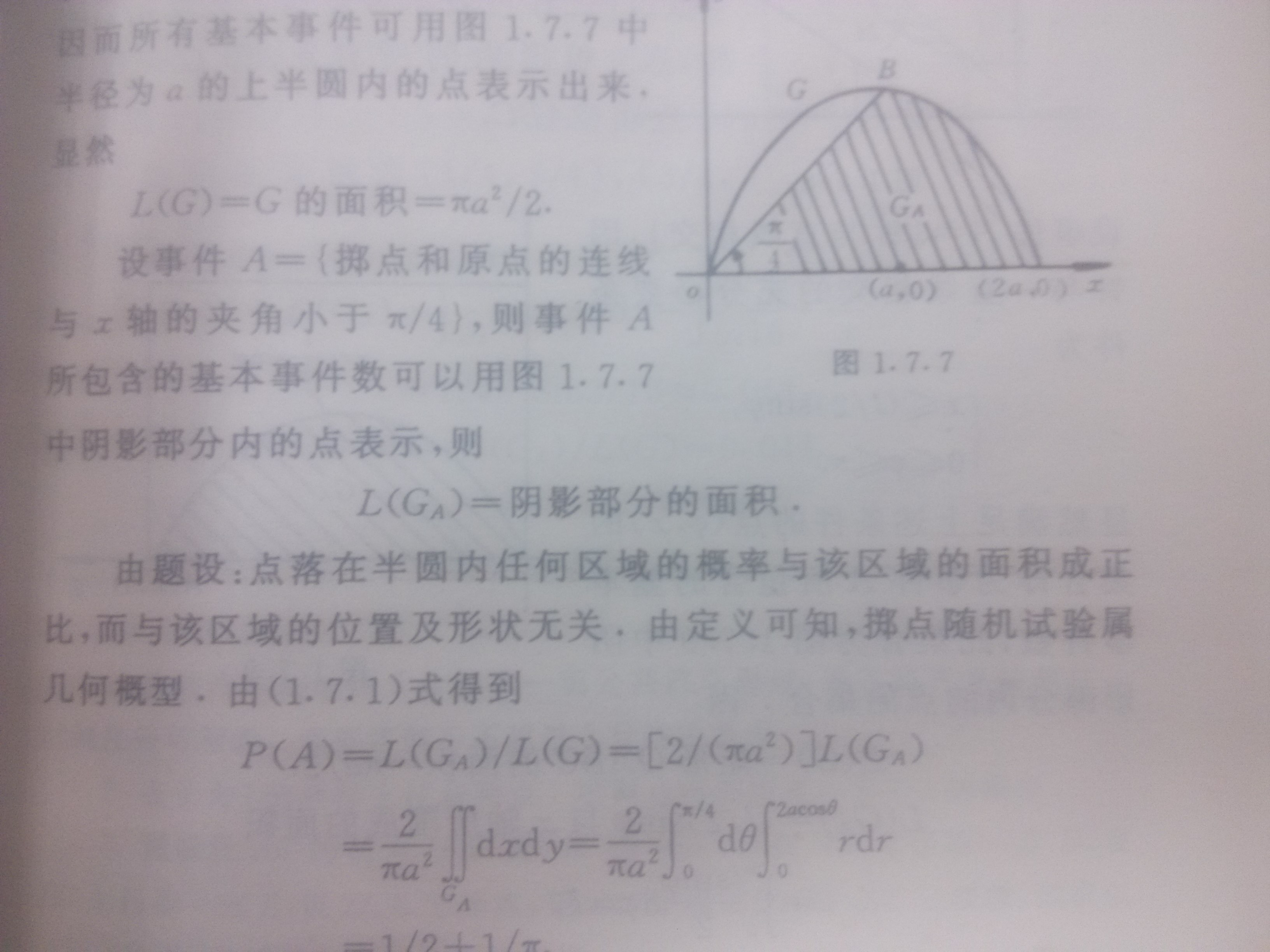需要求出上图中阴影部分的面积，虽然可以通过其他方式求出，但是解答中的二重积分形式显然更具备通用型。

化简式中 dxdy 转化成极坐标表达形式的时候变成了 rdr dΘ    这个过程我无法理解

在查询了相关资料之后确认我的疑问在于二重积分坐标变化的过程

拿出微积分的相关教材 我找了这个公式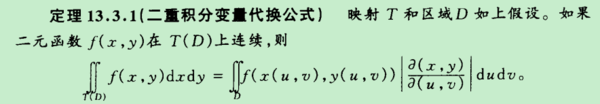（下面的公式源自二阶雅可比行列式， 等式右侧为行列式）

其中| ∂(x,y)/∂(u,v) | =  | x 对 u的偏导       x 对v 的偏导|

| y  对 u的偏导     y 对v 的偏导|

直角坐标系转换成极坐标系的时候满足

x  = r cosΘ

y  = r sinΘ

| ∂(x,y)/∂(r,Θ) | = | cosΘ    -rsinΘ|

| sinΘ   rcosΘ |

= r

ps：

在看到这个公式的时候我对Θ r 的顺序存在了疑问，他们的顺序会影响最后的符号，目前 推测最后的积分顺序相关，我们选择先对r积分，然后对Θ积分，所以是这样的顺序，这一点目前还待验证 。（目前已确认，Θ r 存在关联关系，如果交换积分顺序，那么对应积分中的范围数值也应该相应调整）

展开全文• matlab二重积分代码目录 DIRECTFN软件包是免费软件，用于准确有效地评估在符合三角形或四边形网格的情况下，由Galerkin MoM表面积分方程公式产生的4-D奇异积分。 DIRECTFN的全数值算法适用于以下应用： 弱而强烈的...
• matlab二重积分代码SpinDoctor工具箱 SpinDoctor是一个软件包，可对原型进行扩散磁共振成像（dMRI）的数值模拟。 可以使用SpinDoctor 解决Bloch-Torrey偏微分方程（BTDPE）以获得dMRI信号（工具箱提供了一种稳健地...
• 有了格林公式后，我们在进行环路积分后就不必用老办法了，而是采用二重积分的形式，这样可以大大简化计算步骤 面积分 面有两种表达形式： z=f(x,y)      and    ...
格林公式内容如下：
$\oint_C(F_xdx+F_ydy)=\iint_R(\frac{\partial F_y}{\partial x}-\frac{\partial F_x}{\partial y})$
在之前的文章中，我们讲到过路径积分的方式，然而每次用参数表达x,y后再计算总有些麻烦
有了格林公式后，我们在进行环路积分后就不必用老办法了，而是采用二重积分的形式，这样可以大大简化计算步骤
比如一个圆，原先我们需要先找到它的参数表达式，但现在我们可以直接根据原式来计算了
面积分
面有两种表达形式：
$z=f(x,y)\ \ \ \ \ \ and\ \ \ \ \ \ g(x,y,z)=0$
参数形式
在对线进行积分的时候，我们常用一个参数t来表示x,y
在对面进行积分时，我们则常用两个参数u,v来表示面的三个参数x,y,z
通过u,v,我们可以找到一个面的法向量
$N=r_u×r_v$
$r_u=\partial r/\partial u$
$r_v=\partial r/\partial v$
如果一个面可以被表达为：$f(x,y,z)=0$ 的形式，则可以得到这个面的法向量为：$grad\ f$


展开全文• 其中，z=z(x,y)，Dxy是曲面在xoy平面上的投影，整个积分转化为了二重积分 有一些特殊情况，可以不用做投影死算，而是直接使用一些规则物体求面积的公式直接求出 S为面积，上划线X为形心的x坐标 ...
把对面积的曲线积分中，曲线改为曲面，线密度改为面密度，小段弧长改为小块曲面面积，所求的结果即为曲面物体的质量。

计算公式为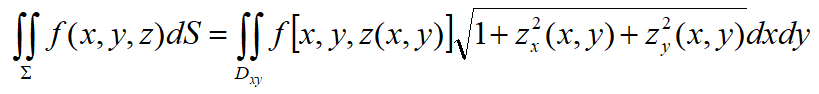其中，z=z(x,y)，Dxy是曲面在xoy平面上的投影，整个积分转化为了二重积分

对称性

若曲面Σ关于xOy对称，则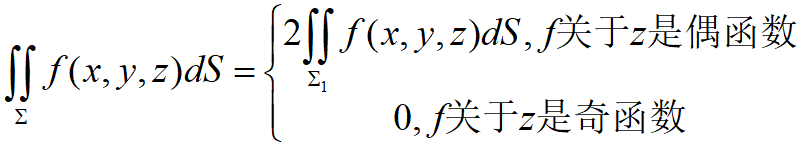其中，Σ1是Σ在xOy上侧的部分。

有一些特殊情况，可以不用做投影死算，而是直接使用一些规则物体求表面积的公式直接求出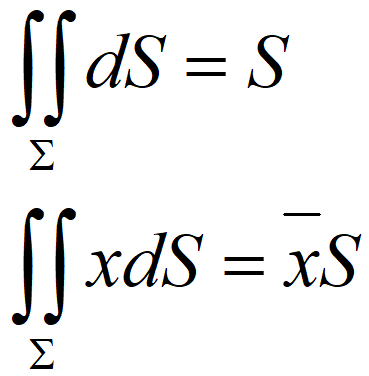S为表面积，上划线X为形心的x坐标


展开全文• 三、常用特殊函数不定积分：四、初等积分方法：五、阿贝尔积分：六、定积分 / 黎曼积分：七、反常积分：八、含参积分：九、二重积分：十、三重积分：十一、曲线积分：十二、曲面积分：十三、场论：十四、关于牛顿-...
积分学：一、不定积分：二、基本初等函数不定积分表：三、常用特殊函数不定积分表：四、初等积分方法：五、阿贝尔积分：六、定积分 / 黎曼积分：七、反常积分：八、含参积分：九、二重积分：十、三重积分：十一、曲线积分：十二、曲面积分：十三、场论：十四、关于牛顿-莱布尼兹公式及其衍生公式的一点思考：

一、不定积分：

概念
释义
性质

原函数
对于函数$f(x)$，若存在同定义域的函数$F(x)$，使得：$F'(x) = f(x)$，则称$F(x)$为$f(x)$的原函数
设$F_0(x)$是$f(x)$的一个原函数，则$F_0(x)+C$也是$f(x)$的原函数

不定积分
函数$f(x)$的全体原函数称为$f(x)$的不定积分，记为$\int f(x)dx$，其中$f(x)$称为积分函数，$f(x)dx$称为积分表达式,$dx$称为积分变量
$\int f(x)dx = F(x)+C$

积分曲线
若$F(x)$是$f(x)$的一个原函数，则把函数图像$y = F(x)$称为$f(x)$的一条积分曲线
$f(x)$的不定积分的所有积分曲线构成积分曲线簇

=> 微分运算和不定积分的关系：
$d[\int f(x)dx] = f(x)dx$
$[\int df(x)] = f(x)+C$
=> 不定积分的性质：
$\int k\cdot f(x)dx = k\cdot \int f(x) dx$
$\int (f(x)\pm g(x))dx = \int f(x) dx \pm \int g(x) dx$
$[\int (f(x)\cdot g(x))dx] ^2 \le [\int f(x) dx]^2 \cdot [\int g(x) dx]^2$（柯西不等式）

二、基本初等函数不定积分表：

函数
不定积分

$f(x) = a$
$F(x) = ax+C$

$f(x) = x^{\alpha}(\alpha \neq -1)$
$F(x) = \frac{1}{\alpha+1}x^{\alpha+1}+C$

$f(x) = a^x$
$F(x) = \frac{1}{\ln a}a^x+C$

$f(x) = \log_a x$
$F(x) = x\log_a x - \frac{x}{\ln a}+C$

$f(x) = sinx$
$F(x) = -cosx+C$

$f(x) = cosx$
$F(x) = sinx+C$

$f(x) = tanx$
$F(x) = -\ln \mid cosx\mid+C$

$f(x) = cotx$
$F(x) = \ln \mid sinx\mid+C$

$f(x) = secx$
$F(x) = ln\mid secx + tanx\mid + C$

$f(x) = cscx$
$F(x) = ln\mid cscx - cotx\mid + C$

$f(x) = arcsinx$
$F(x) = xarcsinx+\sqrt{1-x^2}+C$

$f(x) = arccosx$
$F(x) = xarccosx-\sqrt{1-x^2}+C$

$f(x) = arctanx$
$F(x) = xarctanx-\frac{1}{2}\ln(1+x^2)+C$

三、常用特殊函数不定积分表：

函数
不定积分

$f(x) = \frac{1}{x}$
$F(x) = \ln\mid x\mid+C$

$f(x) = \cfrac{1}{\sqrt{1-x^2}}$
$F(x) = arcsinx + C$

$f(x) = \cfrac{1}{1+x^2}$
$F(x) = arctanx + C$

$f(x) = \cfrac{1}{\sqrt{x^2\pm a^2}}$
$F(x) = ln \mid x+\sqrt{x^2\pm a^2}\mid + C$

$f(x) = e^x$
$F(x) = e^x+C$

$f(x) = \ln x$
$F(x) = x\ln x - x+C$

$f(x) = sec^2 x$
$F(x) = tanx + C$

$f(x) = csc^2 x$
$F(x) = -cotx + C$

$f(x) = shx$
$F(x) = chx+C$

$f(x) = chx$
$F(x) = shx+C$

四、初等积分方法：

1）第一类换元法：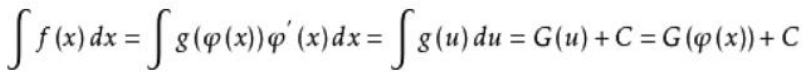2）第二类换元法：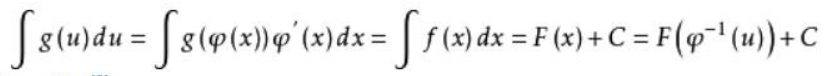3）三角换元法：

含$(a^2-x^2), (x^2+a^2), (x^2-a^2)$等项的积分
一般可以通过分别令：$x = asint,atant,asect$换元转化为有理式积分

含$\sqrt{(x-a)(b-x)}$项的积分 (a<x<b)
一般可以通过令$x = acos^2t+bsin^2t$换元转化为有理式积分

含三角函数的函数$f(sinx,cosx)$的积分
一般可以通过万能公式代换，令：$t = tan\frac{x}{2}$换元转化为有理式积分

含三角函数$sin^ax\times cos^bx$的积分：
若$a$,$b$其中之一为奇数，可以令：$t = cosx$ (a为奇数)或 $t= sinx$ (b为奇数)；
若$a$,$b$都是奇数/偶数，可以令：$t = tanx$
=> 或者可以直接通过分部积分得到递推公式进行求解

4）双曲换元法：

含$(a^2-x^2), (x^2+a^2), (x^2-a^2)$等项的积分
一般可以通过分别令：$x = atht,asht,acht$换元转化为有理式积分

5）倒代换法：

当有理分式的分母的次数较高而分子形式又较简单时
可以尝试通过令：$x = \frac{1}{t}$将其转化为形式更为简单的有理分式积分

6）根式替换法：

含一次分式的根式的函数$f(x,(\frac{ax+b}{cx+d})^{s_1},(\frac{ax+b}{cx+d})^{s_2},..,(\frac{ax+b}{cx+d})^{s_n})$
一般可以通过令：$t = (\frac{ax+b}{cx+d})^s$，其中$s = [s_1,s_2,...s_n]$将其转化为有理式积分

7）二项式微分的换元法：

含二项式微分的函数$f(z,z^q(a+bz)^p)$
如果$p,q,p+q$之中有一个是整数，则
一般可以通过令：$t = \sqrt[n]{a+bz},或者t = \sqrt[n]{\cfrac{a+bz}{z}}$, 其中$n$是$p$的分母

8）二次根式的欧拉替换法：
含二次根式的函数$f(x,\sqrt{ax^2+bx+c})$：

若$Δ> 0$,可以令：$\sqrt{ax^2+bx+c} = t(x -λ)$ , 其中$λ$为二次式的一实根
若$Δ=0$,可以令：$\sqrt{ax^2+bx+c} = (x -λ)$, 其中$λ$为二次式的唯一实根
若$Δ< 0$ 且$a>0$, 可以令： $\sqrt{ax^2+bx+c} = t -\sqrt{ax}$

9）奇次根式的阿贝尔替换法：

含奇次根式分式的函数$f(x,\cfrac{1}{(ax^2+bx+c)^{\frac{2n+1}{2}}})$
可以考虑令：$t = \cfrac{ax+\frac{b}{2}}{\sqrt{ax^2+bx+c}}$

10）二次根式的部分分式法：
含二次根式的函数$f(x,\sqrt{ax^2+bx+c})$
按照分解定律一定可以被化成三种类型的积分：

$\int\cfrac{P(x)}{\sqrt{ax^2+bx+c}}dx$：利用待定系数法，可以令：
$\int\cfrac{P(x)}{\sqrt{ax^2+bx+c}} dx= Q(x)\sqrt{ax^2+bx+c}+\lambda \int \cfrac{dx}{\sqrt{ax^2+bx+c}}$
其中$Q(x)$是比$P(x)$低一次的多项式，$λ$为常数

$\int\cfrac{A}{(x-\alpha)^k\sqrt{ax^2+bx+c}}dx$：利用倒变换，可以令：
$(x-\alpha) = \frac{1}{t}$将该类型化为上种类型

$\int\cfrac{Ax+B}{(x^2+px+q)^k\sqrt{ax^2+bx+c}}dx$：利用分式线性替换，可以令：
$x = \cfrac{ct+d}{t+1}$，选择合适的$c,d$将原积分化为：$\int \cfrac{P(t)dt}{(t^2+s)^k\sqrt{\alpha t^2+\beta}}$的形式，然后再继续分解化为若干个$\int \cfrac{A_itdt}{(t^2+s)^{k_i}\sqrt{\alpha t^2+\beta}}$和$\int \cfrac{B_idt}{(t^2+s)^{k_i}\sqrt{\alpha t^2+\beta}}$，再分别利用倒变换和阿贝尔变换求解即可

11）分部积分法：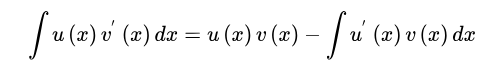12）  有理函数积分：
根据实系数多项式分解定理：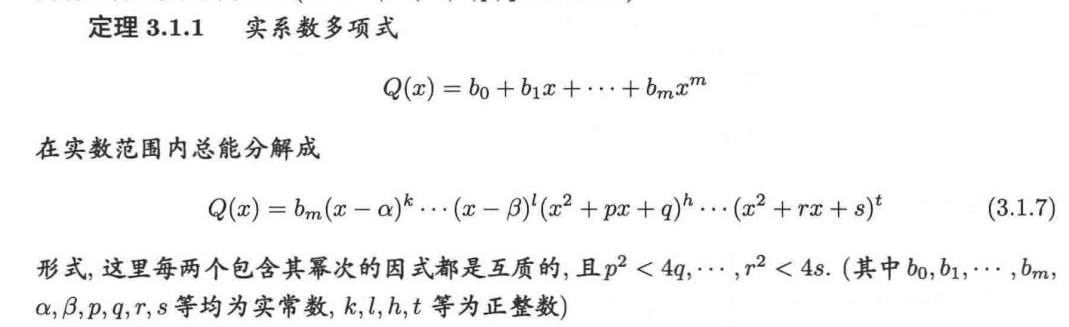因此所有有理函数只需要研究去除整式部分后的真分式再分解为的最简分式，如下所示：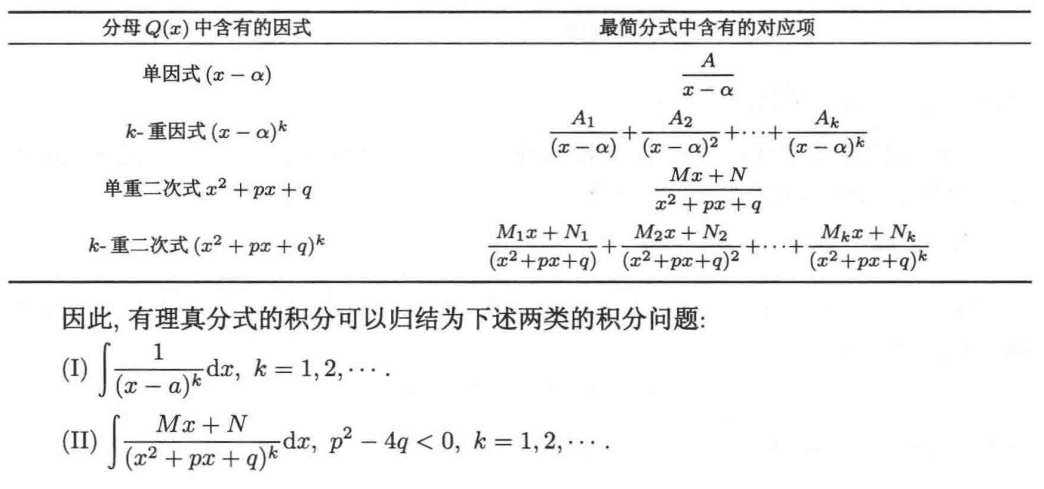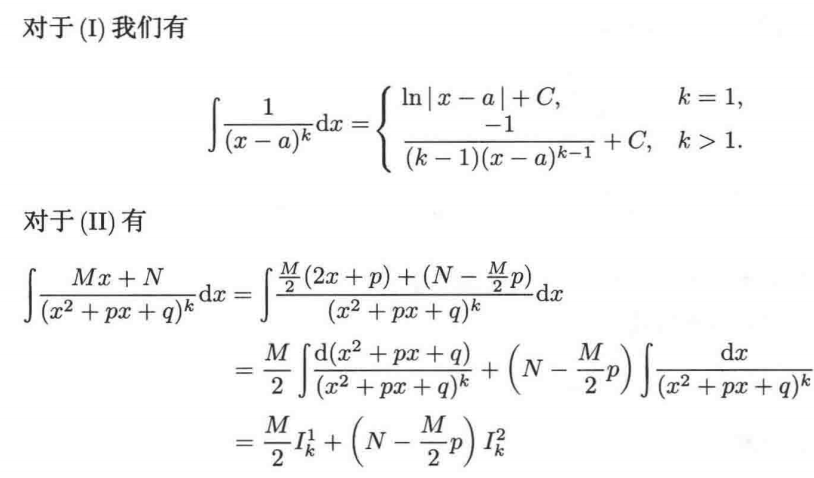分别讨论$I_k^1$和$I_k^2$的解法：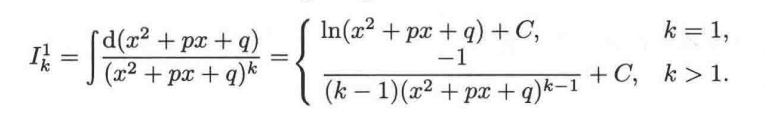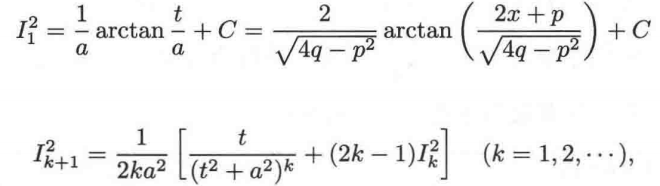=> 有理式分解方法：

待定系数法：设出所有可能的部分分式，再通分求解系数；

奥氏方法：直接通过分离积分的:
有理部分（有理部分的分母一定是各个部分分式分母的次高次项的乘积，分子利用待定系数）;
无理部分（无理部分的导数的分母一定是各个部分分式分母的一次项的乘积，分子利用待定系数）;
然后再通分求解系数

五、阿贝尔积分：
1）定义：形如$f(x,y)$的函数的积分（其中$y$是$x$的代数函数，即满足代数方程$P(x,y) = 0$）叫做阿贝尔积分，例如$f(x,\sqrt{x^2+px+q})$；
2）有限形状表示定理：阿贝尔积分是否可以表示成有限形状，主要以$P(x,y)=0$决定的曲线的性质所决定，若该曲线能够用参数方程$x = x(t),y = y(t)$表示，其中$x(t),y(t)$是有理函数，则对应的阿贝尔积分一定能够在有限形状中求得；
3）椭圆积分：一般形如$f(x,\sqrt{ax^4+bx^3+cx^2+dx+e})$的函数的积分，不能在有限形状中求出的，称为椭圆积分
=> 任何椭圆积分可分解成三类标准椭圆积分：
Ⅰ：$F(k,z) = \int \cfrac{dz}{ \sqrt{(1-z^2)(1-k^2z^2)}} \xrightarrow{z=sinx} F(k,x) = \int \cfrac{dx}{ \sqrt{1-k^2sin^2x}}$
Ⅱ：$E(k,z) = \int \cfrac{z^2dz }{ \sqrt{(1-z^2)(1-k^2z^2)}}\xrightarrow{z=sinx} E(k,x) = ∫ \sqrt{1-k^2sin^2x}dx$
Ⅲ：$\Pi(k,h,z) = \int \cfrac{dz}{ (1+hz^2)\sqrt{(1-z^2)(1-k^2z^2)}} \xrightarrow{z=sinx}\Pi(k,h,x) =\int \cfrac{dx}{(1+hsin^2x)\sqrt{(1-k^2sin^2x)}}$

六、定积分 / 黎曼积分：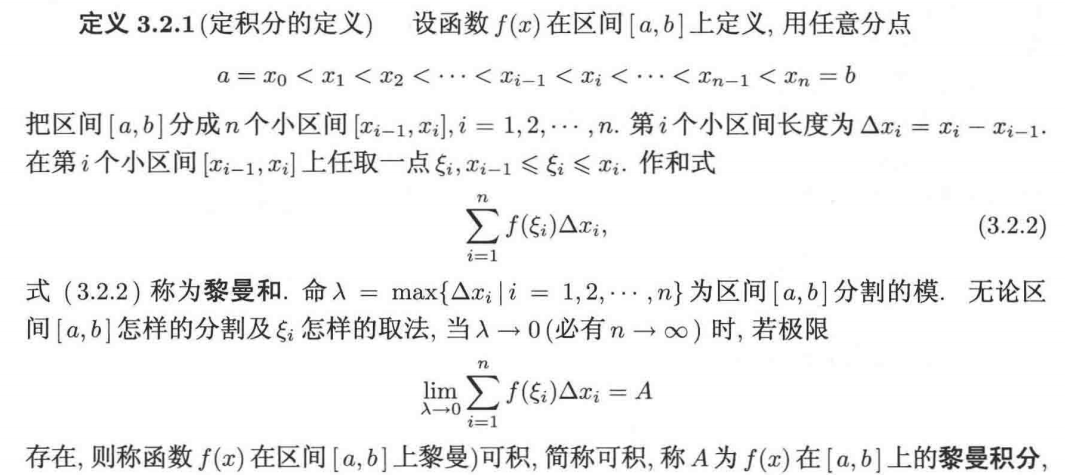=> 函数可积的必要条件和常见类型：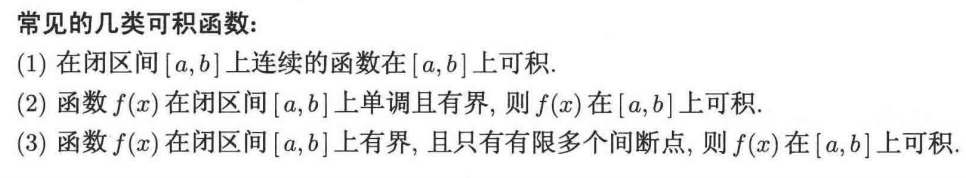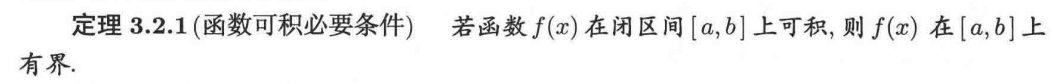=> 可积函数的性质：
1）函数$f(x)$在$[a,b]$上可积，则函数$|f(x)|$在$[a,b]$上可积；
2）可积性对于加法、减法和乘法运算封闭；
3）函数$f(x)$在$[a,b]$上可积，则$f(x)$在$[a,b]$的任一子区间上可积；
4）改变可积函数在有限个点上的值，既不会破坏其可积性，也不会改变原定积分的值
=> 定积分运算性质：
1）$\int_a^bf(x)dx = \int_a^cf(x)dx + \int_c^bf(x)dx$；
2）$|\int_a^bf(x)dx| \le\int_a^b|f(x)|dx$ ；
3）柯西不等式：$(\int_a^bf(x)g(x)dx)^2 \le \int_a^bf^2(x)dx\times\int_a^bg^2(x)dx$
4）牛顿-莱布尼兹公式：
$\int_a^bf(x)dx = F(b) - F(a)$，其中$F(x)$为$f(x)$的原函数
5）积分中值定理：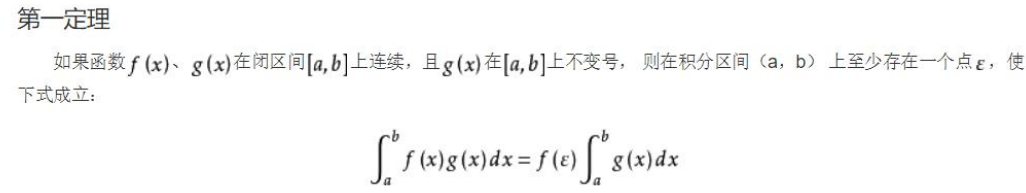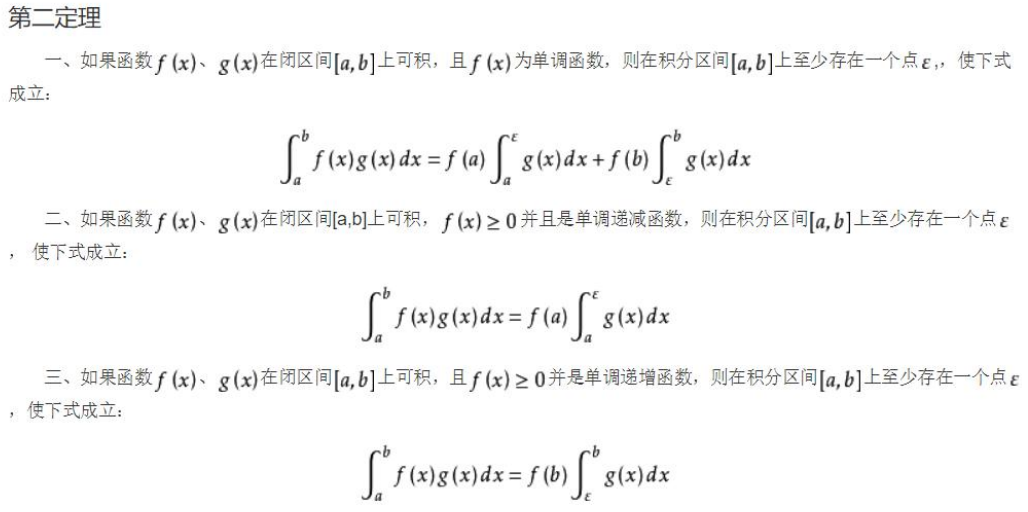七、反常积分：
1）两种类型：
无穷区间上：$\int_a^{+\infty}f(x)dx = \lim_{b\rightarrow+∞}\int_a^bf(x)dx$；
无界函数：$\int_a^bf(x)dx = \lim_{\varepsilon\rightarrow 0}\int_a^{b-\varepsilon}f(x)dx$，
其中上限$b$为$f(x)$的奇点（下限为奇点可类似定义）
2）牛顿-莱布尼兹公式：
无穷区间上：$\int_a^{+\infty}f(x)dx = F(+∞) - F(a)$
无界函数： $\int_a^bf(x)dx = \lim_{\varepsilon\rightarrow 0}[F(b-\varepsilon) - F(a)]$
3）敛散性判定定理：
Ⅰ. 比较定理：
至少从某处$x(x>a)$开始时不等式$f(x)\le g(x)$恒成立，则:
从$\int_a^{+∞}g(x)dx$收敛，知$\int_a^{+∞}f(x)dx$收敛；
从$\int_a^{+∞}f(x)dx$发散，知$\int_a^{+∞}g(x)dx$发散 ；
=> 令$K = \lim\cfrac{f(x)}{g(x)}$, 则当$0时，两函数保持相同的敛散性；
Ⅱ. 柯西判别法：
=> 设x充分大时，可令$f(x) = \cfrac{g(x)}{x^λ}(λ> 0)$，则：
若$λ> 1$且$g(x)\le c<+∞$，则$\int_a^{+∞}f(x)dx$收敛；
若$λ\le 1$且$g(x)\ge c>0$，则$\int_a^{+∞}f(x)dx$发散；
=> 设x充分靠近b，可令$f(x) = \cfrac{g(x)}{ (b-x)^λ}(λ> 0)$，则：
若$λ< 1$且$g(x)\le c<+∞$，则$\int_a^{b}f(x)dx$收敛；
若$λ\ge 1$且$g(x)\ge c>0$，则$\int_a^{b}f(x)dx$发散；
Ⅲ. 阿贝尔判别法：
若$f(x)$在$[a,+∞]$可积，且$g(x)$在区间内单调有界，则：
积分$\int_a^{+∞}f(x)g(x)dx$收敛
Ⅳ. 狄里克雷判别法：
若$\int_a^{b}f(x)dx$在任何有限区间$[a,b]$可积且有界，且$g(x)$在区间内单调趋近于零，则：
积分$\int_a^{+∞}f(x)g(x)dx$收敛

八、含参积分：

1）欧拉积分：
Ⅰ. 第一型欧拉积分（$B$函数）：
$B(x,y) = \int_0^1 t^{x-1}(1-t)^{y-1}dt$
=> 性质：
$B(x,y) = B(y,x)$
$B(x,y) = \cfrac{\Gamma(x)\Gamma(y)}{\Gamma(x+y)}$
Ⅱ. 第二型欧拉积分（$Γ$函数）：
$\Gamma(x) = \int_0^{+\infty}e^{-t}t^{x-1}dt$
=> 性质：
$\Gamma(x+1) = x\Gamma(x)$，特殊地，当$x = n \in N$时，$\Gamma(n+1) = n!$
$\Gamma(x)\Gamma(1-x) = \cfrac{\pi}{sin\pi x}(0，此性质称为余元公式
2）变限积分：（仅以变上限积分的定义为例，其他情况类似）=> 变限积分求导法则：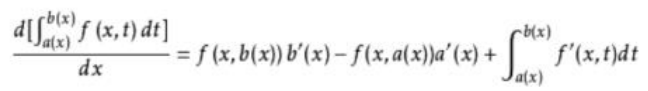九、二重积分：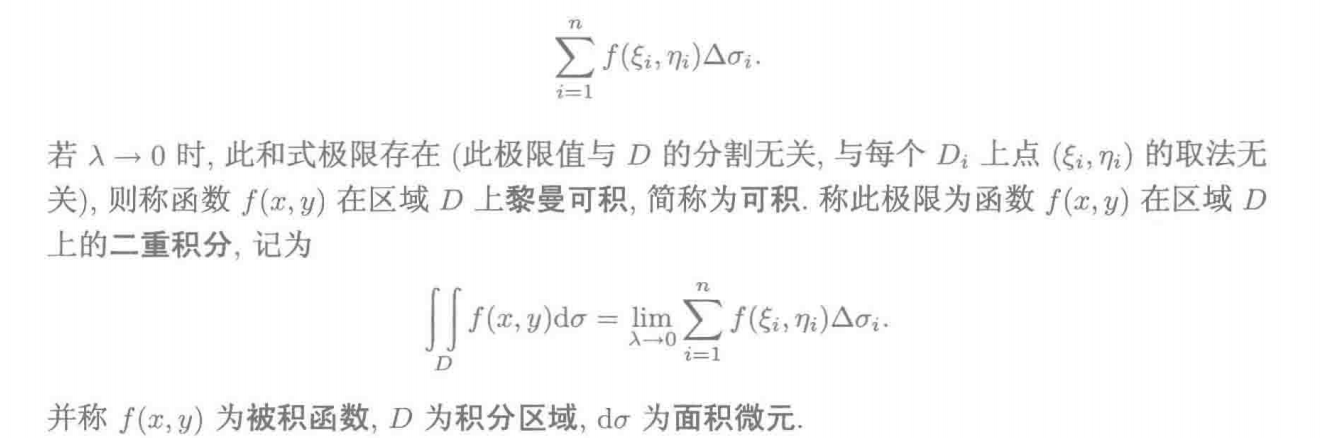=> 二重积分中值定理：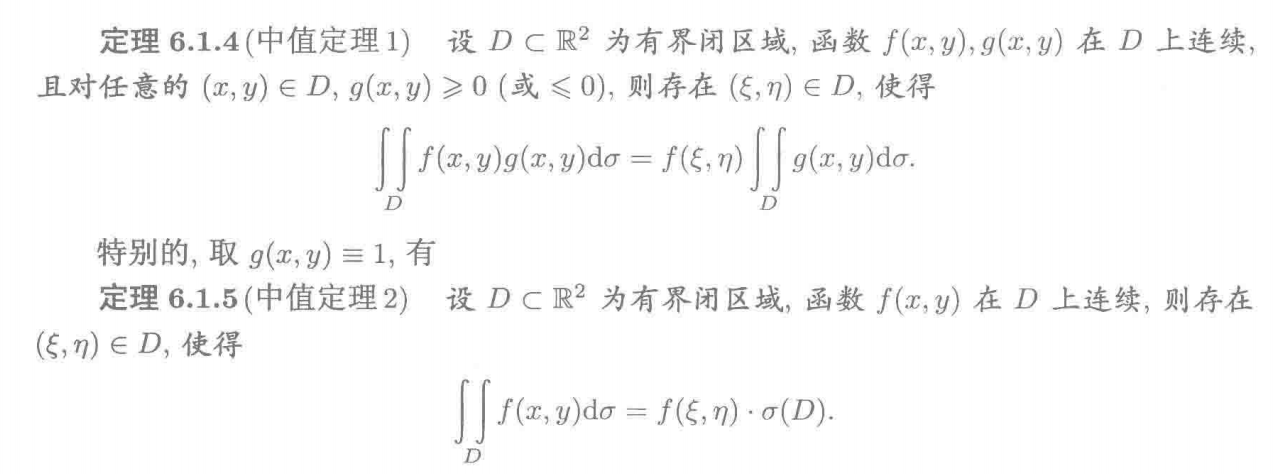=> 二重积分计算方法：
① 累次积分法：（仅以先对y积分，再对x积分为例，反之类似）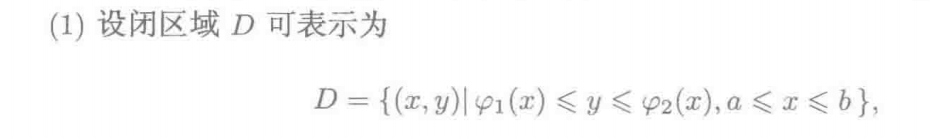则：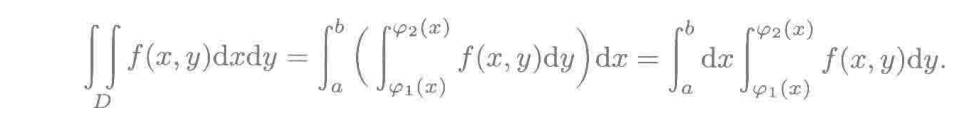② 换元积分法：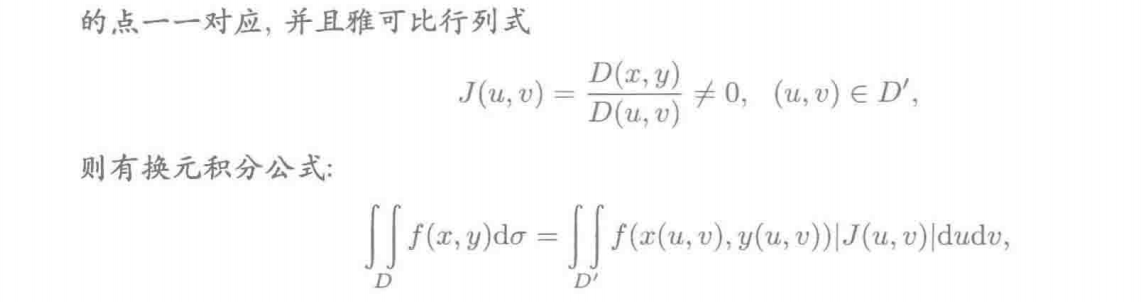常见地有：
极坐标变换：若$x = \rho cos\theta,y = \rho sin\theta$，则：
$dxdy = |\cfrac{D(x,y) }{ D(ρ,θ)}|dρdθ = ρdρdθ$
广义极坐标变换：若$x = a\rho cos\theta,y = b\rho sin\theta$，则：
$dxdy = |\cfrac{D(x,y) }{ D(ρ,θ)}|dρdθ = abρdρdθ$

十、三重积分：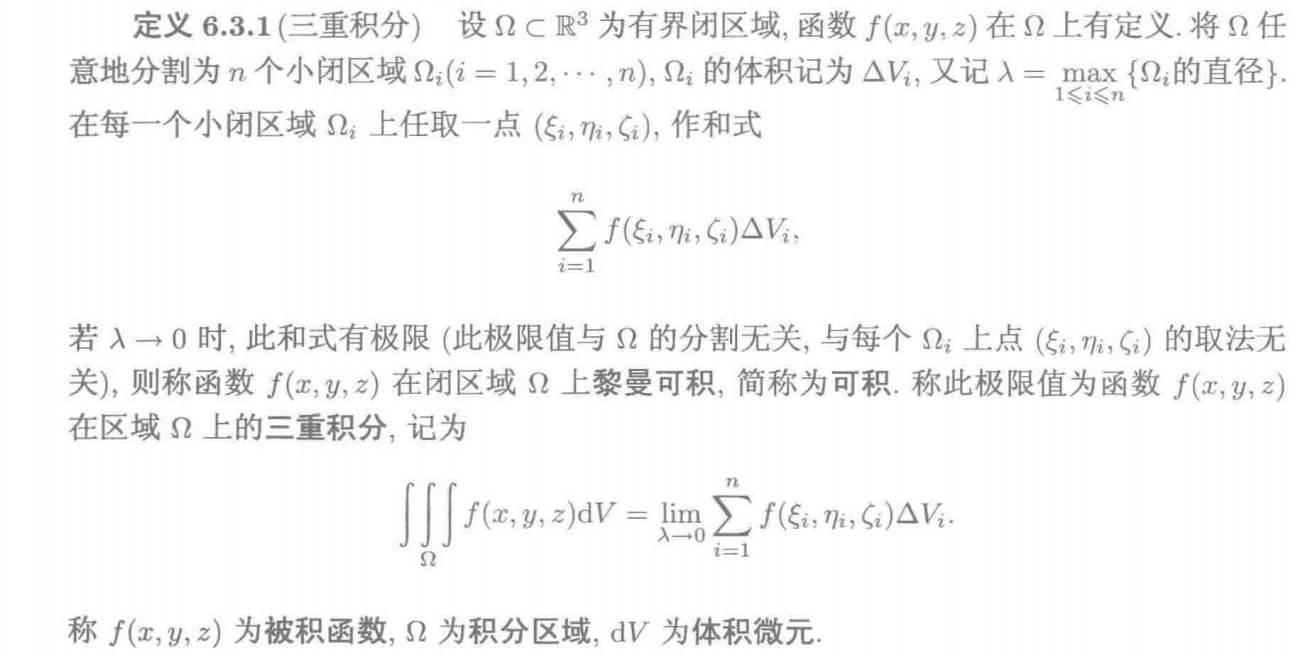=> 二重积分计算方法：
① 累次积分法：
先对积分区域任意一条平行于z轴的直线进行积分，再对xy投影面进行积分：（简称先一后二）
设：则：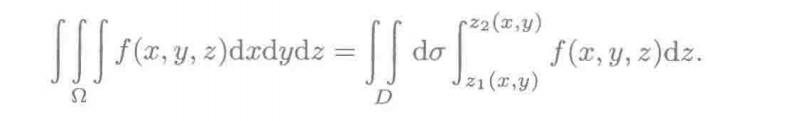先对积分区域任意一个z轴的法切面进行积分，再对z轴进行积分：（简称先二后一）
设：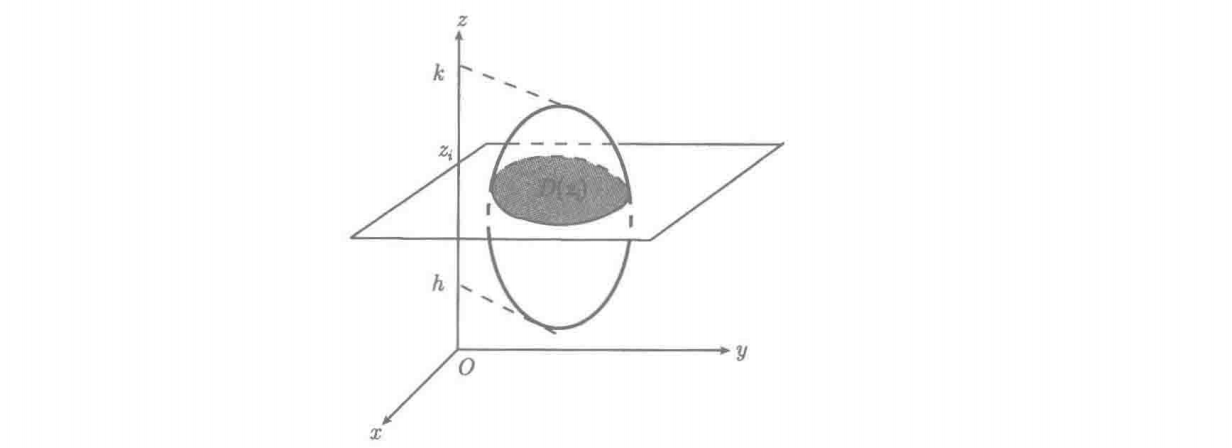则：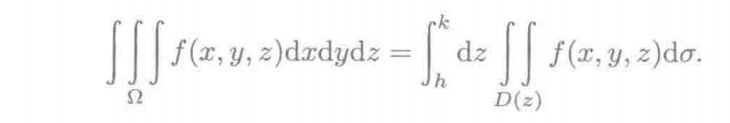② 换元积分法：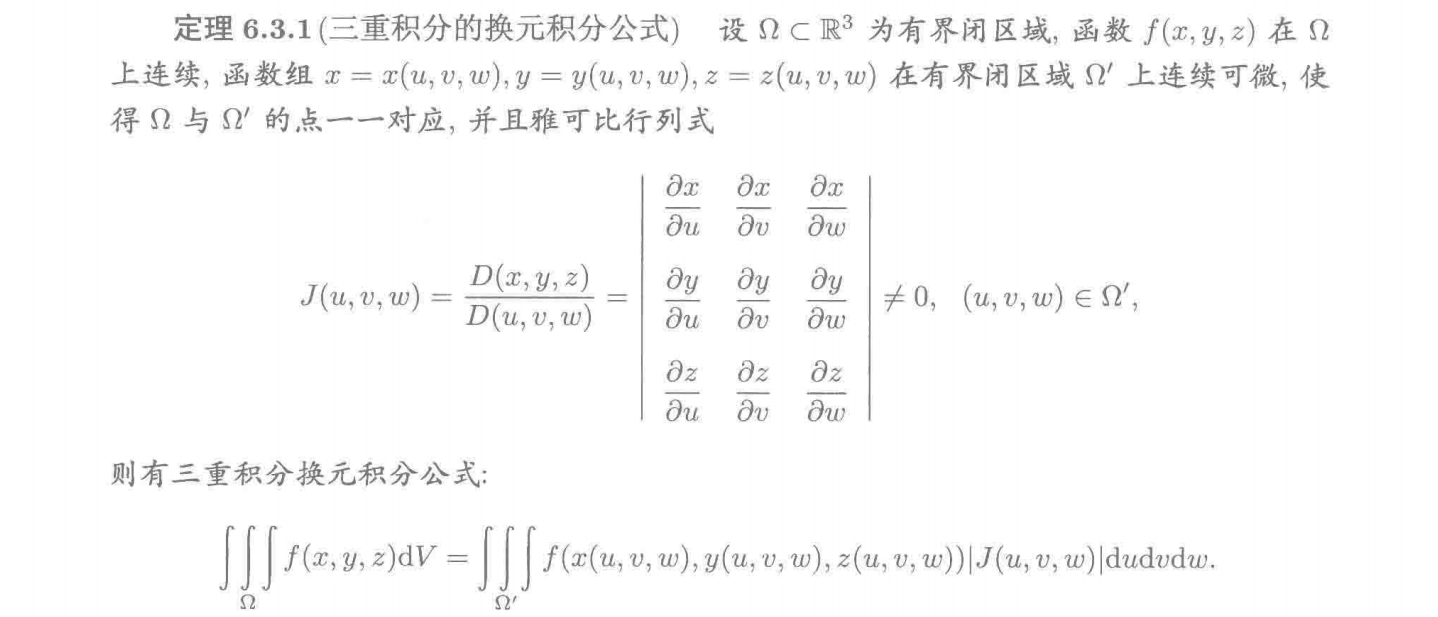常见地有：
柱坐标变换：若$x = \rho cos\theta,y = \rho sin\theta,z=z$，则：
$dxdydz = |\cfrac{D(x,y,z) }{ D(ρ,θ,z)}|dρdθdz = ρdρdθdz$
球坐标变换：若$x = r cos\theta sin\varphi,y = r sin\theta sin\varphi,z = r cos\varphi$，则：
$dxdydz = |\cfrac{D(x,y,z) }{ D(r,\theta,\varphi)}|drdθd\varphi = r^2sin\varphi drdθd\varphi$
广义球坐标变换：若$x = ar cos\theta sin\varphi,y = br sin\theta sin\varphi,z = cr cos\varphi$，则：
$dxdydz = |\cfrac{D(x,y,z) }{ D(r,\theta,\varphi)}|drdθd\varphi = abcr^2sin\varphi drdθd\varphi$

十一、曲线积分：
1）第一类曲线积分（对弧长的曲线积分）：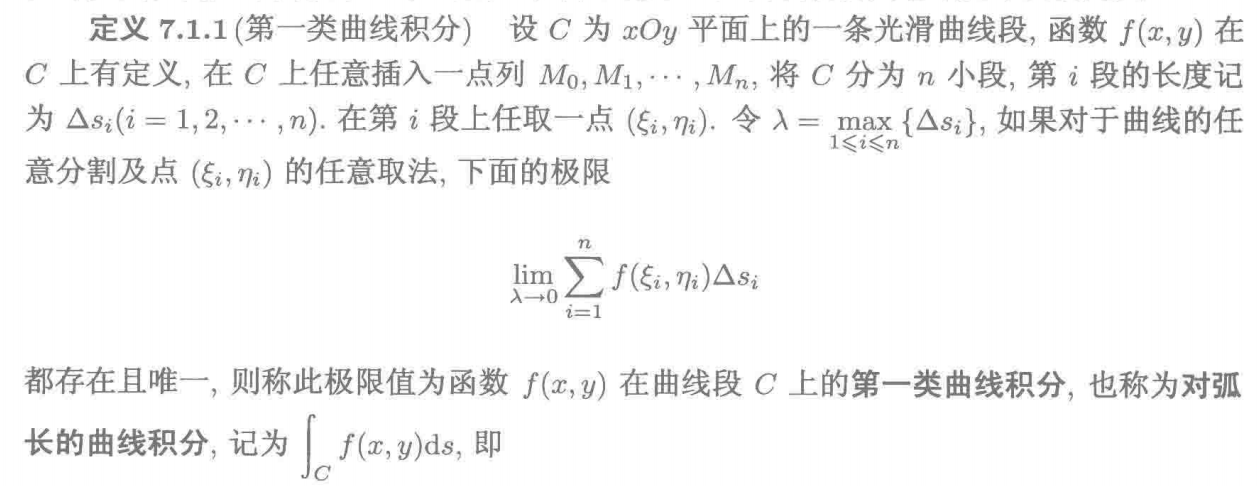=> 无向性：第一类曲线积分不依赖于积分曲线的走向，即：$\int_{AB}fds = \int_{BA}fds$
=> 对平面曲线积分的计算公式：
① 直角坐标：$∫_Cf(x,y)ds = ∫_a^bf(x,y)\sqrt{1+(y'_x)^2} dx$
② 参数坐标：$∫_Cf(x,y)ds =∫_{t_1}^{t_2}f(x(t),y(t))\sqrt{x'(t)^2+y'(t)^2} dt$
③ 极坐标：$∫_Cf(x,y)ds = ∫_{θ1}^{θ_2}f(rcosθ,rsinθ)\sqrt{r(θ)^2+r'(θ)^2}dθ$
=> 对空间曲线积分的计算公式：
① 参数坐标：$∫_Cf(x,y,z)ds =∫_{t_1}^{t_2}f(x(t),y(t),z(t))\sqrt{x'(t)^2+y'(t)^2+z'(t)^2} dt$
② 直角坐标 / 极坐标：一般需要化为参数坐标，再代入公式①进行计算
2）第二类曲线积分：（对坐标的曲线积分）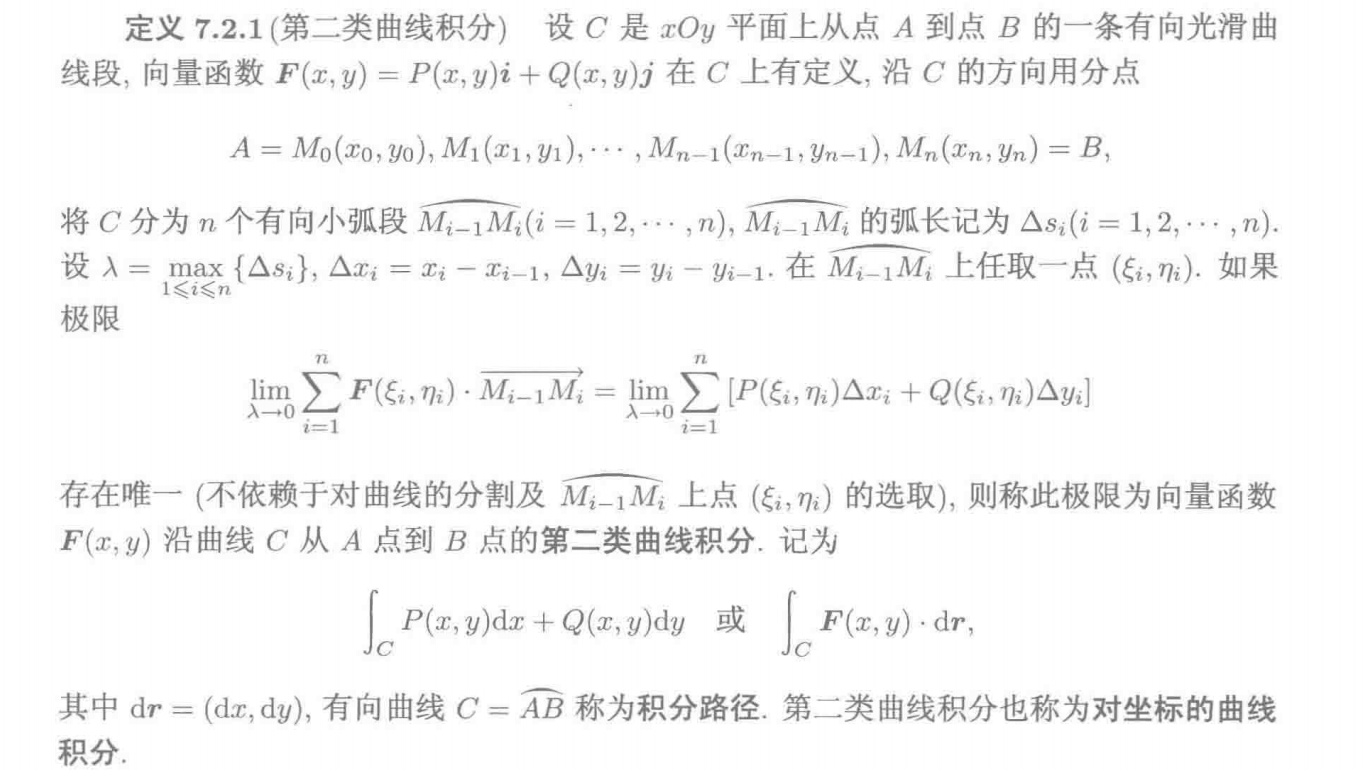=> 有向性：第二类曲线积分依赖于积分曲线的走向，且有：$\int_{AB}fds = -\int_{BA}fds$
=> 对平面曲线积分的计算公式：
① 直角坐标：$∫_CP(x,y)dx+Q(x,y)dy = ∫_C[P(x,y(x)) + Q(x,y(x))y'(x)]dx$
② 参数坐标：$∫_CP(x,y)dx+Q(x,y)dy = ∫_{t_1}^{t_2}[P(x(t),y(t))x'(t)+Q(x(t),y(t))y'(t)]dt$
③ 极坐标：一般化为参数坐标或直角坐标，再代入公式②或①进行计算
=> 对空间曲线积分的计算公式：
① 参数坐标：$∫_CP(x,y,z)dx+Q(x,y,z)dy+R(x,y,z)dz =∫_{t_1}^{t_2}[P(x(t),y(t),z(t))x'(t)+Q(x(t),y(t),z(t))y'(t)+R(x(t),y(t),z(t))z'(t) ]dt$
② 直角坐标 / 极坐标：一般需要化为参数坐标，再代入公式①进行计算
3）两类曲线积分的转化：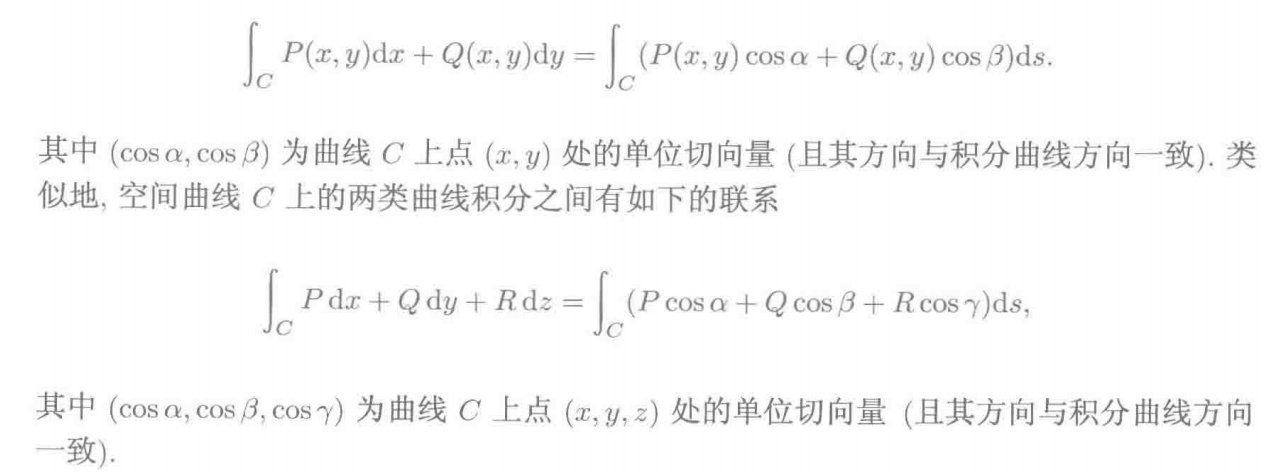4）曲线积分与积分路径无关的充要条件：
=> 对于平面曲线积分：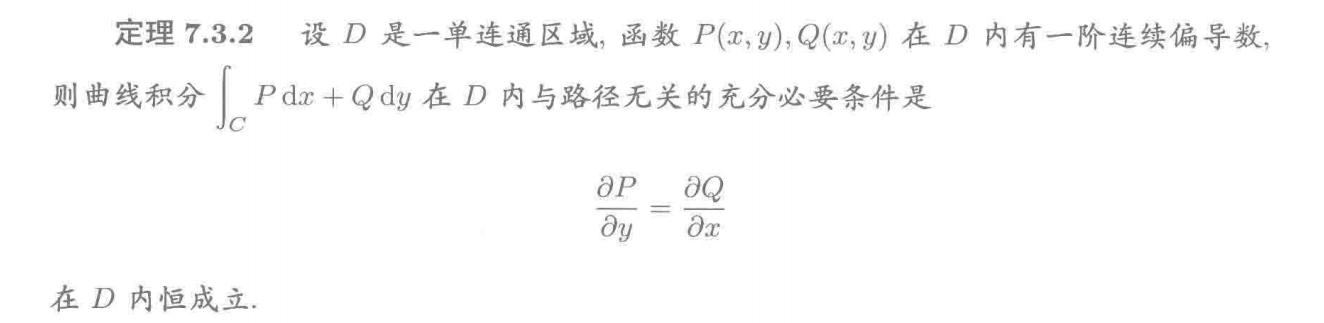=> 对于空间曲线积分：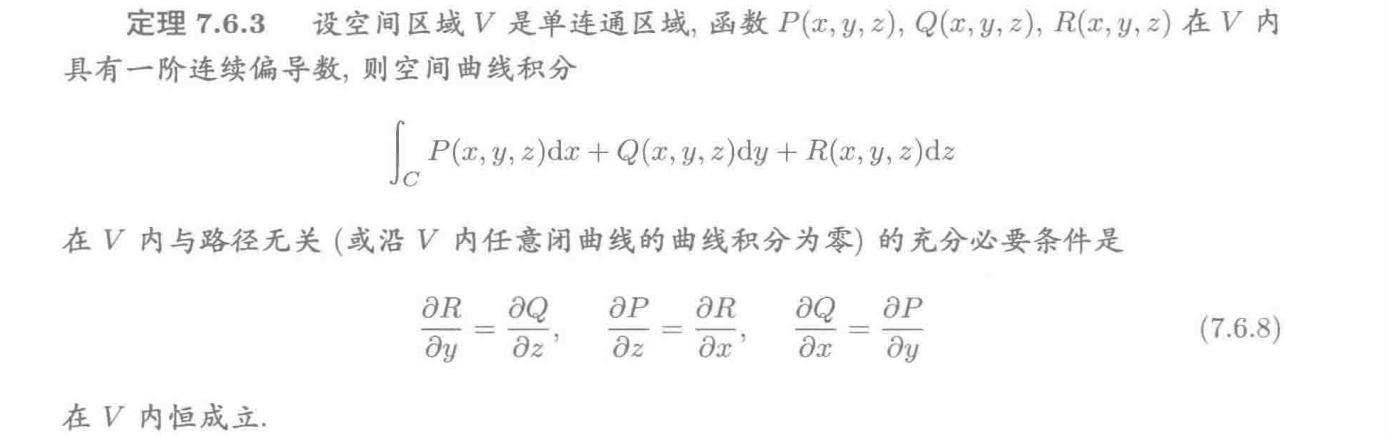5）对平面曲线积分的格林公式：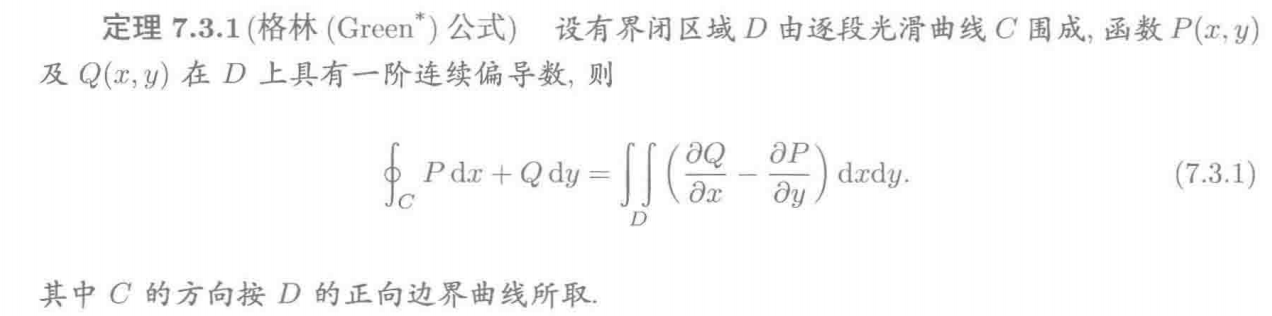=> 格林公式是二重积分和平面曲线积分之间的连接桥梁，也可看作“二维版”的牛顿-莱布尼兹公式
6）对空间曲线积分的斯托克斯公式：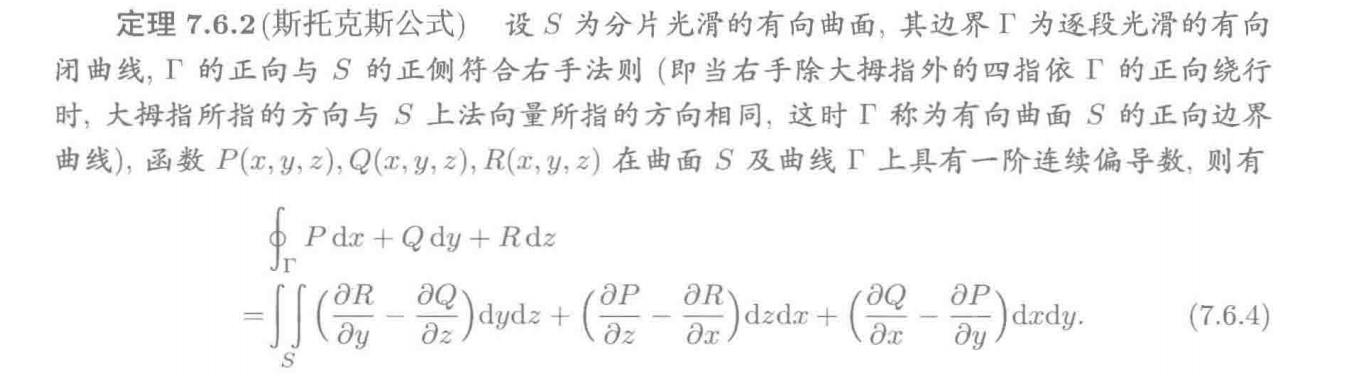另有行列式写法：=> 斯托克斯公式是曲面积分和空间曲线积分之间的连接桥梁，也可看作“弯曲版”的格林公式

十二、曲面积分：

1）第一类曲面积分：（对曲面面积的积分）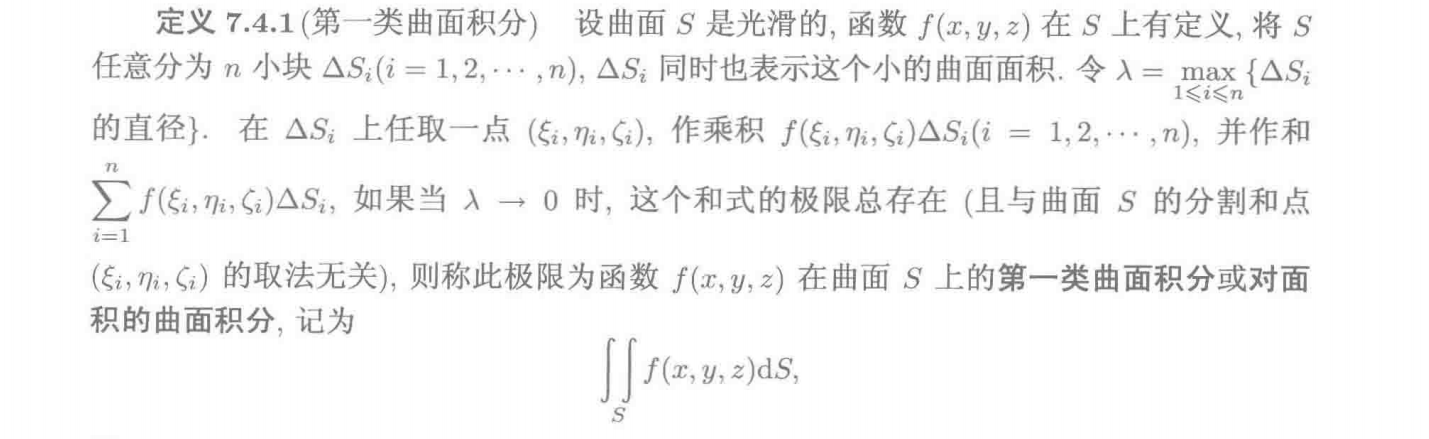=> 第一类曲面积分的计算公式：
① 参数方程：$\iint_Sf(x,y,z)dS = \iint_{D_{uv}}f(x(u,v),y(u,v),z(u,v))\sqrt{EG-F^2}dudv$
其中：$E = r_u'\cdot r_u', G = r_v'\cdot r_v', F = r_u'\cdot r_v'$，$r = (x(u,v),y(u,v),z(u,v))$
② 直角方程：$\iint_Sf(x,y,z)dS =\iint_{D_{xy}}f(x,y,z(x,y))\sqrt{1+z_x'^2+z_y'^2}dxdy$
2）第二类曲面积分：（对坐标平面的曲面积分）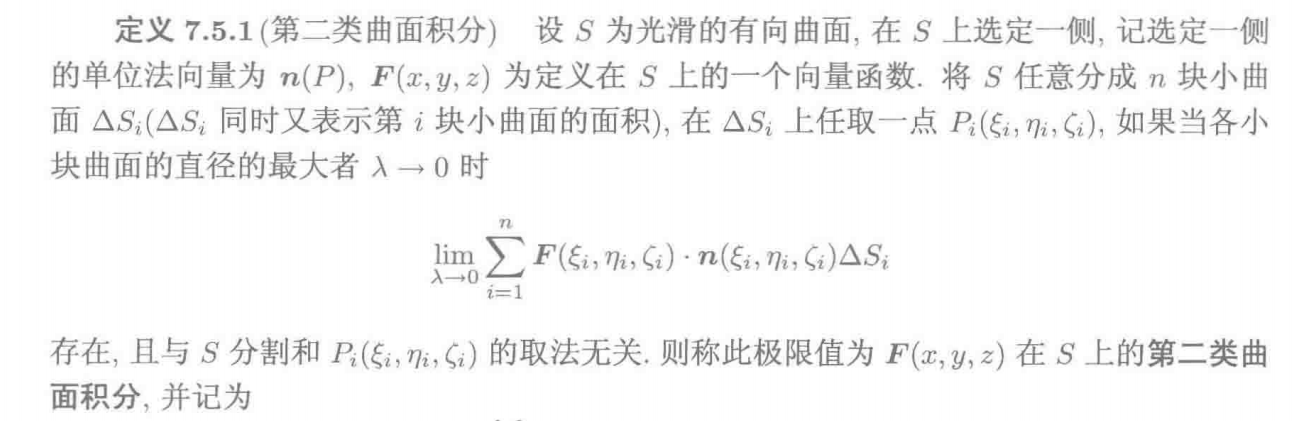=> 第二类曲面积分的计算公式：
① 参数方程：$\iint_SP(x,y,z)dydz+Q(x,y,z)dzdx+R(x,y,z)dxdy = \iint_{D_{uv}}[PA+QB+RC]dudv$
其中：$(A,B,C) = r_u'\times r_v'$，$r = (x(u,v),y(u,v),z(u,v))$
② 直角方程：$\iint_SP(x,y,z)dydz+Q(x,y,z)dzdx+R(x,y,z)dxdy =\pm\iint_{D_{xy}}[P(-z_x')+Q(-z_y')+R]dxdy$
其中的正负号有S的定向决定，法向量指向上侧时为正，反之为负（仅以$\rm XY$平面为坐标投影面为例；同理，若以$\rm XZ$平面为坐标投影面，则法向量指向右侧为正，反之为负；若以$\rm YZ$平面为坐标投影面，则法向量指向前侧为正，反之为负）
3）两类曲面积分的转化：4）对曲面积分的高斯公式：（“三维版的格林公式”）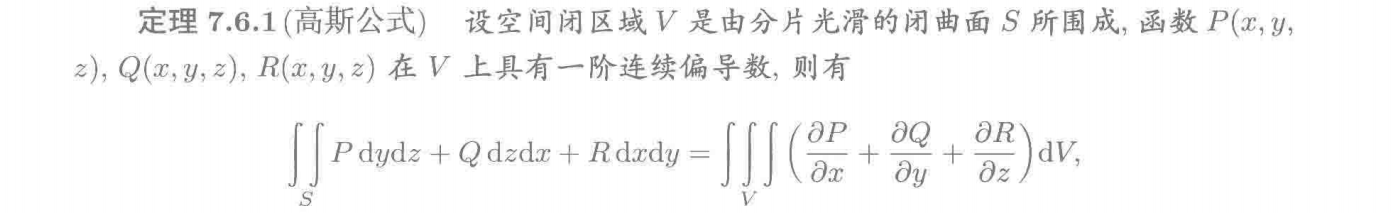=> 高斯公式是三重积分和曲面积分之间的连接桥梁，也可看作“三维版”的格林公式

十三、场论：

1）数量场的梯度：
考虑数量场$u = f(x,y,z)$: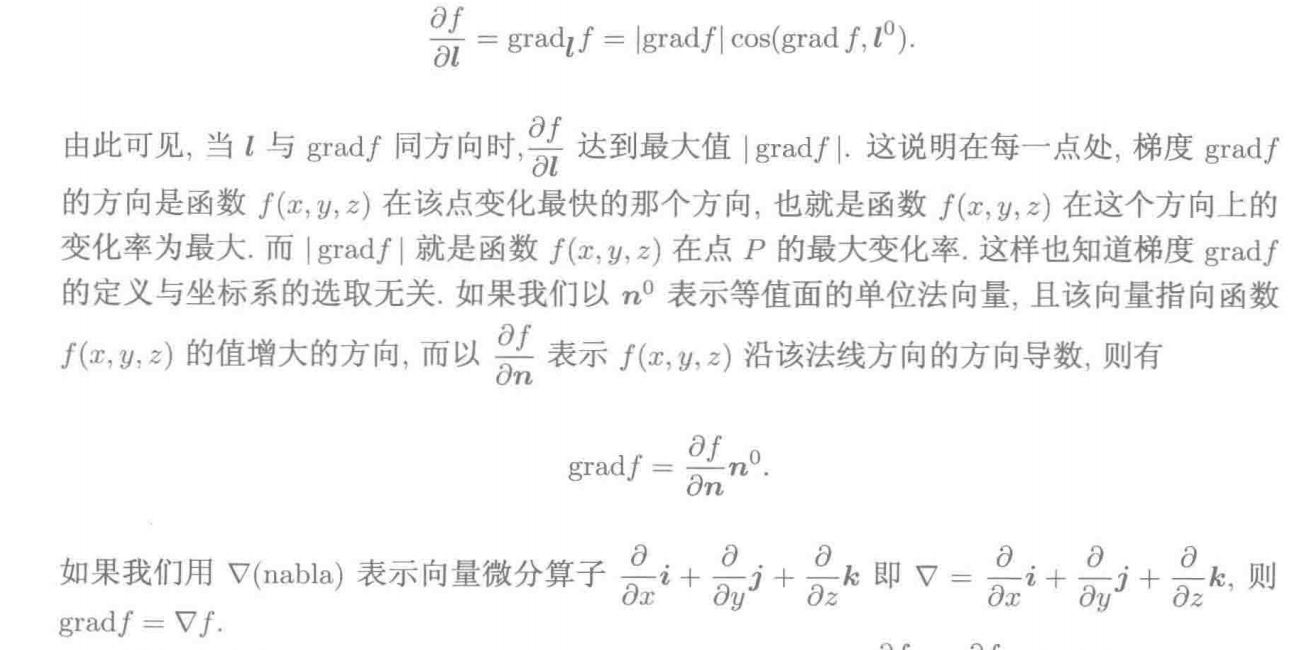2）向量场的散度：3）向量场的旋度：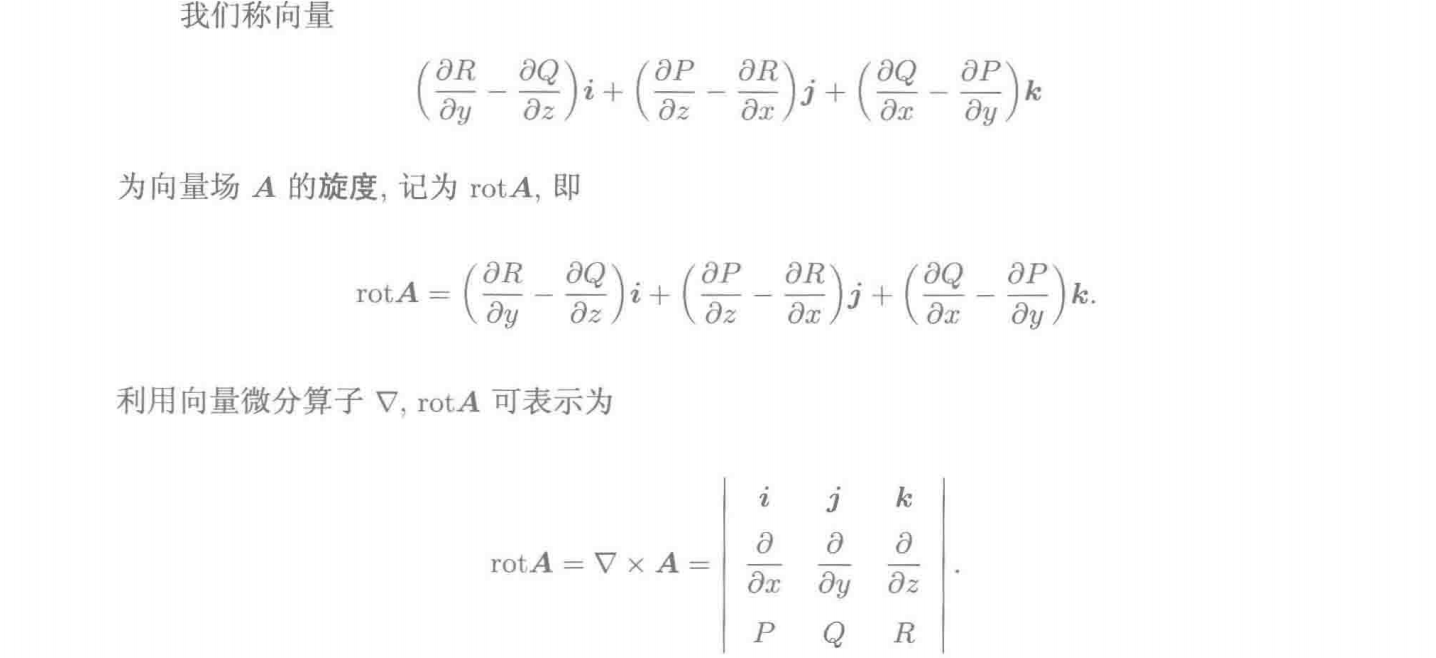4）场论形式的斯托克斯公式：
$\oint_C Adr = \iint_S rot A\cdot n dS$，其中：$n = (cos\alpha,cos\beta,cos\gamma)$
5）场论形式的高斯公式：
$\oiint_S A\cdot ndS = \iiint_V div A dV$，其中：$n = (cos\alpha,cos\beta,cos\gamma)$

十四、关于牛顿-莱布尼兹公式及其衍生公式的一点思考：
经读者思考，我们其实可以从某个角度将牛顿-莱布尼兹公式、格林公式、斯托克斯公式、高斯公式统一起来，即：
它们均表达的是将若干个函数对某一个有界几何区域上的积分转化为该若干个函数的原函数或偏原函数对该区域的边界上的积分，例如：
① 牛顿-莱布尼兹公式：将一维线段上的积分$\int_a^b f(x)dx$转化为其原函数对该一维线段的两端点上的积分$F(x)|^b_a$；
② 格林公式：将二维单连通平面上的积分$\iint_D (\cfrac{\partial Q}{\partial x}-\cfrac{\partial P}{\partial y})dxdy$转化为其偏原函数对该二维平面的闭合边界曲线上的积分$\oint_C Pdx+Qdy$；
③ 斯托克斯公式：将二维单连通曲面上的积分$\iint_S [(\cfrac{\partial R}{\partial y}-\cfrac{\partial Q}{\partial z})dydz+(\cfrac{\partial P}{\partial z}-\cfrac{\partial R}{\partial x})dzdx+(\cfrac{\partial Q}{\partial x}-\cfrac{\partial P}{\partial y})dxdy]$转化为其偏原函数对该二维曲面的闭合边界曲线上的积分$\oint_C Pdx+Qdy+Rdz$；
④ 高斯公式：将三维闭区域上的积分$\iiint_V [\cfrac{\partial P}{\partial x}+\cfrac{\partial Q}{\partial y}+\cfrac{\partial R}{\partial z}]dxdydz$转化为其偏原函数对该三维区域的闭合边界曲面上的积分$\oiint_S Pdydz+Qdzdx+Rdxdy$；


展开全文• 格林公式表达了平面闭区域上的二重积分与其边界曲线上的曲线积分之间的关系,而高斯公式表达了空间闭区域上的三重积分与其边界曲面上的曲面积分之间的关系，这个关系可陈述如下： 【定理】设空间闭区域是由分片光滑...高等数学
• 4.1.2二重积分上、下限的确定——穿线法 4.1.3几个典型的积分次序及积分限变换的例子 4.1.4两个一元函数乘积的积分 4.2三重积分 4.2.1三重积分的定义 4.2.2三重积分的傅比尼定理 4.2.3三重积分的算例 4.3重积分的...
• 4.1.2二重积分上、下限的确定——穿线法 4.1.3几个典型的积分次序及积分限变换的例子 4.1.4两个一元函数乘积的积分 4.2三重积分 4.2.1三重积分的定义 4.2.2三重积分的傅比尼定理 4.2.3三重积分的算例 4.3重积分的...
• 二重积分 偏导数 偏积分 下面列出一些上面所需的基本公式 导数 多元函数偏导数和偏积分 对于函数z=f(x,y)的导数 求函数对x的导数，把y当作常数k，不参与导数运算 求函数对y的导数，把x当作常数k，不参与...
• 格林公式的有效性　通过上章的内容，我们知道格林公式有两种表达： 尽管物理意义不同，但数学上是相同的，都是把线积分转换为R区域的二重积分。格林公式的成立有个前提条件——向量场需要在R区域处处有定义，更严格...单连通区域
• •----第十六章 二重积分 --------§1.二重积分的定义及简单性质 ------------586.柱形长条体积的问题 ------------587.化二重积分为逐次积分 ------------588.二重积分的定义 ------------589.二重积分存在的条件...
• 1·3 常用初等函数的不定积分公式 1·4 有理函数的积分法 1·5 无理函数的积分法 1·6 超越函数的积分法 1·7 各种函数的不定积分的例题 2.定积分 2·1 有理整函数的定积分 2·2 定积分 2·3 定积分的基本性质 2·4 ...
• 1·3 常用初等函数的不定积分公式 1·4 有理函数的积分法 1·5 无理函数的积分法 1·6 超越函数的积分法 1·7 各种函数的不定积分的例题 2.定积分 2·1 有理整函数的定积分 2·2 定积分 2·3 定积分的基本性质 2·4 ...
• 3.4.1 利用定积分的微分元素法化二重积分为累次积分 3.4.2 二重积分的微分元素法 3.4.3 利用二重积分的微分元素法计算曲面面积 第四章 级数及其应用 4.1 数项级数 4.1.1 数项级数及其收敛 4.1.2 正项级数的收敛判别...
• 4． 求导公式表 5． 函数导数的四则运算 2.不同类型函数的求导法则及高阶导数 1． 复合函数的求导法则 2． 隐函数的求导法则 3． 参数方程所确定的函数的求导法则 4． 高阶导数 3.函数的微分及应用 1． 微分的定义 2...
• 二重积分：\iint_{D}f(x,y)dxdy 三重积分：\iiint_{\Omega}f(x,y,z)dxdydz 函数极限：\lim\limits_{x\to0}f(x)=0 求和符号:\sum 加下标是_,上标是^ ex: \sum_i ^n为 ∑in\sum_i^n∑in​ \iint_{D}^n为 ∬Dn\iint_D^n...latex
• 我们向Noether势添加Wald型计数器二重形式，并将守恒全局电荷定义为表面积分。 我们针对各种字符串背景（几何和非几何）检查O（D，D）协变量公式。 在所有情况下，我们都发现与先前的结果完全吻合。 我们的公式不仅...
• 二重三角积分 §2. 关于二重三角和的不等式 §3. Titchmarsh关于*的估计 §4. 二重三角和的另一种估计方法 第十五章Goldbach-ВИНОГРАДОВ定理 §1. 引论 §2. 证明的?要步骤 §3. 基本区间上的积分 §4. ...
• 莱布尼兹公式和变上限积分函数的求导； ; margin-right:0cm">4．掌握定积分的微元法，掌握用定积分来表达面积、体积、弧长，了解功、水压力； ; margin-right:0cm">  ; margin-...人工智能 自然语言处理
• 5.4.3 Zip（x）多项式的微分公式、递推关系式和积分公式 5.5 第二类复数柱多项式定义及其递推公式 5.6 第三类复数柱多项式定义及其递推公式 5.7 复数柱多项式函数渐近展开 5.8 其他边界条件下的圆域非稳态热传导问题...